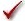## Please enable JavaScript to view this site.

 Navigation: PRINCIPLES OF STATISTICS > Analysis checklists Analysis checklist: One-way ANOVA

One-way ANOVA compares the means of three or more unmatched groups. Read elsewhere to learn about choosing a test, and interpreting the results.

###Are the populations distributed according to a Gaussian distribution?

One-way ANOVA assumes that you have sampled your data from populations that follow a Gaussian distribution. While this assumption is not too important with large samples due to the Central Limit Theorem, it is important with small sample sizes (especially with unequal sample sizes). Prism can test for violations of this assumption, but normality tests have limited utility.

If your data do not come from Gaussian distributions, you have three options. Your best option is to transform the values (perhaps to logs or reciprocals) to make the distributions more Gaussian. Another choice is to use the Kruskal-Wallis nonparametric test instead of ANOVA. A final option is to use ANOVA anyway, knowing that it is fairly robust to violations of a Gaussian distribution with large samples.

###Do the populations have the same standard deviation?

One-way ANOVA assumes that all the populations have the same standard deviation (and thus the same variance). This assumption is not very important when all the groups have the same (or almost the same) number of subjects, but is very important when sample sizes differ.

InStat tests for equality of variance with two tests: The Brown-Forsythe test and Bartlett's test. The P value from these tests answer this question: If the populations really have the same variance, what is the chance that you'd randomly select samples whose variances are as different from one another as those observed in your experiment. A small P value suggests that the variances are different.

Don't base your conclusion solely on these tests. Also think about data from other similar experiments. If you have plenty of previous data that convinces you that the variances are really equal, ignore these tests (unless the P value is really tiny) and interpret the ANOVA results as usual. Some statisticians recommend ignoring tests for equal variance altogether if the sample sizes are equal (or nearly so).

In some experimental contexts, finding different variances may be as important as finding different means. If the variances are different, then the populations are different -- regardless of what ANOVA concludes about differences between the means.

###Are the data unmatched?

One-way ANOVA works by comparing the differences among group means with the pooled standard deviations of the groups. If the data are matched, then you should choose repeated-measures ANOVA instead. If the matching is effective in controlling for experimental variability, repeated-measures ANOVA will be more powerful than regular ANOVA.

###Are the “errors” independent?

The term “error” refers to the difference between each value and the group mean. The results of one-way ANOVA only make sense when the scatter is random – that whatever factor caused a value to be too high or too low affects only that one value. Prism cannot test this assumption. You must think about the experimental design. For example, the errors are not independent if you have six values in each group, but these were obtained from two animals in each group (in triplicate). In this case, some factor may cause all triplicates from one animal to be high or low.

###Do you really want to compare means?

One-way ANOVA compares the means of three or more groups. It is possible to have a tiny P value – clear evidence that the population means are different – even if the distributions overlap considerably. In some situations – for example, assessing the usefulness of a diagnostic test – you may be more interested in the overlap of the distributions than in differences between means.

###Is there only one factor?

One-way ANOVA compares three or more groups defined by one factor. For example, you might compare a control group, with a drug treatment group and a group treated with drug plus antagonist. Or you might compare a control group with five different drug treatments.

Some experiments involve more than one factor. For example, you might compare three different drugs in men and women. There are two factors in that experiment: drug treatment and gender. These data need to be analyzed by two-way ANOVA, also called two factor ANOVA.

###Is the factor “fixed” rather than “random”?

Prism performs Type I ANOVA, also known as fixed-effect ANOVA. This tests for differences among the means of the particular groups you have collected data from. Type II ANOVA, also known as random-effect ANOVA, assumes that you have randomly selected groups from an infinite (or at least large) number of possible groups, and that you want to reach conclusions about differences among ALL the groups, even the ones you didn't include in this experiment. Type II random-effects ANOVA is rarely used, and Prism does not perform it.

###Do the different columns represent different levels of a grouping variable?

One-way ANOVA asks whether the value of a single variable differs significantly among three or more groups. In Prism, you enter each group in its own column. If the different columns represent different variables, rather than different groups, then one-way ANOVA is not an appropriate analysis. For example, one-way ANOVA would not be helpful if column A was glucose concentration, column B was insulin concentration, and column C was the concentration of glycosylated hemoglobin.